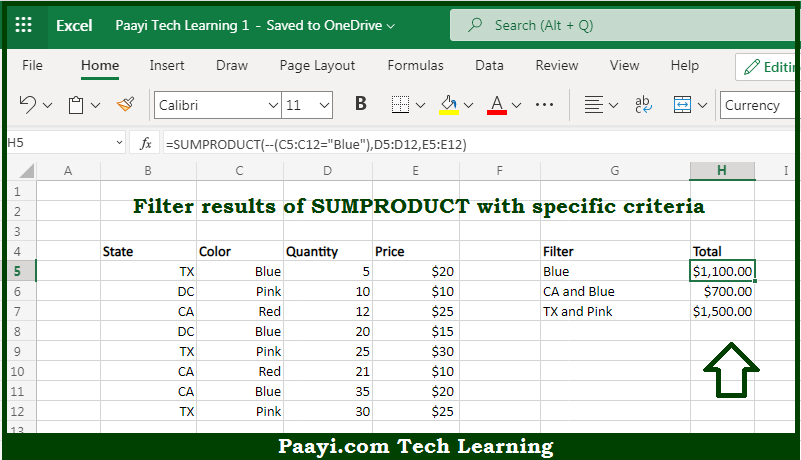# Learn How to SUMPRODUCT With IF in Microsoft Excel

Written by | 0 Comments | 425 Views

In this article, you will learn how to SUM various things in Microsoft Excel using a single/combination(s) of functions. You will also know how to SUMPRODUCT With IF and see the generic formula.

SUMPRODUCT With IF in Microsoft Excel

The main purpose of this formula is to filter the results of SUMPRODUCT with specific criteria. Here we will learn how to SUMPRODUCT with the IF function in the workbook in Microsoft Excel. That implies, with the help of a formula based on the SUMPRODUCT function you can able to filter results of SUMPRODUCT with specific criteria. So, with the help of this formula, you can able to SUMPRODUCT with the IF function in the workbook in Microsoft Excel.

General Formula to SUMPRODUCT With IF

=SUMPRODUCT(expression, range)

The Explanation for the SUMPRODUCT With IFSo we know that with the help of the given formula above you can able filter results of SUMPRODUCT with specific criteria. Here we will learn how to SUMPRODUCT with the IF function in the workbook in Microsoft Excel. As we know that the example provided here demonstrates one of the key strengths of the SUMPRODUCT function. And that is the ability to filter data with basic logical expressions instead of the IF function. It should be noted that Inside SUMPRODUCT, the first array is a logical expression to filter on the provided color. So, with the help of this formula, you can able to SUMPRODUCT with the IF function in the workbook in Microsoft Excel.